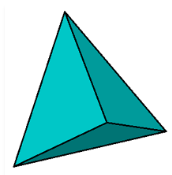# What is a solid triangle called

In geometry, a solid that is made up of four triangular faces is called tetrahedron. Also, a pyramid that has its base, triangle in shape is called a triangular pyramid. See the diagram below to understand.Solid figures are those figures that have surface area and volume. But a triangle is a plane figure which has only area. Therefore, we cannot state a triangle as a solid.(2)(0)